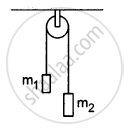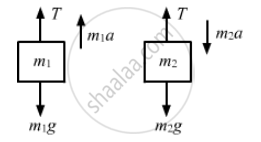# In a Simple Atwood Machine, Two Unequal Masses M1 and M2 Are Connected by a String Going Over a Clamped Light Smooth Pulley. in a Typical Arrangement (Figure 5−E7), M1 = 300 G and M2 = 600 G - Physics

Sum

In a simple Atwood machine, two unequal masses m1 and m2 are connected by a string going over a clamped light smooth pulley. In a typical arrangement (In the following figure), m1 = 300 g and m2 = 600 g. The system is released from rest. (a) Find the distance travelled by the first block in the first two seconds; (b) find the tension in the string; (c) find the force exerted by the clamp on the pulley.#### Solution

The masses of the blocks are m1 = 0.3 kg and m2 = 0.6 kg
The free-body diagrams of both the masses are shown below:For mass m1,
T − m1g = m1a    ...(i)
For mass m2,
m2g − T= m2a    ...(ii)

Adding equations (i) and (ii), we get:
g(m2 − m1) = a(m1 + m2)
$\Rightarrow a = g{\left( \frac{m_2 - m_1}{m_1 + m_2} \right)}$
$= 9 . 8 \times \frac{0 . 6 - 0 . 3}{0 . 6 + 0 . 3}$
$= 3 . 266 m/ s^2$

(a) t = 2 s, a = 3.266 ms−2, u = 0
So, the distance travelled by the body,
$S = ut + \frac{1}{2}a t^2$
$= 0 + \frac{1}{2}\left( 3 . 266 \right) 2^2 = 6 . 5 m$

(b) From equation (i),
T = m1 (g + a)
= 0.3 (3.8 + 3.26) = 3.9 N

(c)The force exerted by the clamp on the pulley,
F = 2T = 2 × 3.9 = 7.8 N

Concept: Newton’s Second Law of Motion
Is there an error in this question or solution?

#### APPEARS IN

HC Verma Class 11, Class 12 Concepts of Physics Vol. 1
Chapter 5 Newton's Laws of Motion
Q 22 | Page 80